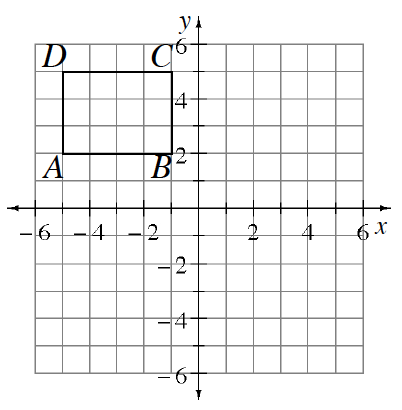### Home > GB8I > Chapter 3 Unit 4 > Lesson INT1: 3.2.1 > Problem3-81

3-81.

Copy rectangle $ABCD$ at right on graph paper.

1. Rotate rectangle $ABCD \:90º$ counterclockwise $(\circlearrowleft )$ about the origin to create rectangle $A^\prime B^\prime C^\prime D^\prime$. Name the coordinates of $C^\prime$.

2. Reflect rectangle $ABCD$ across the vertical line $x = 1$ to create rectangle $A^{\prime \prime }B^{\prime \prime }C^{\prime \prime }D^{\prime \prime }$. Name the coordinates of $C^{\prime \prime}$.

3. Translate rectangle $ABCD$ so that $C$ is moved $5$ units down and $12$ units right. Name the coordinates of $C^{\prime \prime \prime }$. How is this transformation related to the line $y=−\frac{5}{12}x+1$?

4. What is the area of rectangle $ABCD$?Use the eTool below to solve each part.
Click the link at right for the full version of the eTool: 3-81 HW eTool